# 2nd PUC Physics Question Bank Chapter 1 Electric Charges and Fields

Students can Download 2nd PUC Physics Chapter 1 Electric Charges and Fields Questions and Answers, Notes Pdf, 2nd PUC Physics Question Bank with Answers helps you to revise the complete Karnataka State Board Syllabus and to clear all their doubts, score well in final exams.

## Karnataka 2nd PUC Physics Question Bank Chapter 1 Electric Charges and Fields

### 2nd PUC Physics Electric Charges and Fields One Marks Questions and Answers

Question 1.
Define charge on a body.
Charge is that electric property on a body by virtue of which it attracts a body of opposite kind of charge and repels a body of like kind of charge.

Question 2.
Mention the SIU of electric charge. (March 2014)
The SIU of electric charge is coulomb (C).

Question 3.
What is the nature of charge carried by an electron?
Negative.Question 4.
What is the value of quantised unit of charge or what is the smallest unit of charge?
1.6 × 1019C

Question 5.
Mention the value of charge on an electron.
Charge on an electron is 1.6 × 10-19C’

Question 6.
Mention the value of charge on a proton.
Charge on a proton is‘+ 1.6 × 10-19C’.

Question 7.
What is the nature of charge induced on a conductor brought closer to a positively charged body?
Negative charge.

Question 8.
An uncharged conductor is brought in contact with a positively charged body. What is the nature of charge acquired by the conductor?
Positive charge.

Question 9.
Can an insulator be charged by the method of conduction?
No. There are no free electrons in insulators.

Question 10.
Say whether charge on a body varies with speed or not.
Charge on a body is invariant of speed.

Question 11.
What will happen to the mass of a charged body?
Mass of a body charged to + ve decreases and charged to -ve increases.Question 12.
What is the charge on an atomic nucleus?
Charge on an atomic nucleus is +Ze where ‘Z’ is atomic number of an element and e = 11.6 × 10-19C.

Question 13.
A surface encloses + 10 nC and -2 nC of charge. What is the net charge enclosed by it?
The net charge enclosed by the surface in + 8nC.

Question 14.
Is charge a scalar or a vector?
Electric charge is a scalar physical quantity.

Question 15.
Can charge be created?
Charge can neither be created not destroyed. Charge is conserved.

Question 16.
Define Electric field.
The space surrounding the given charge in which a positive test charge experiences a force is known as Electric field.

Question 17.
Define Electric intensity at a point in an electric field.
Electric intensity at a point in an electric field may be defined as the force experienced by a positive test charge placed at that point.

Question 18.
Give the SI unit of Electric field intensity.
NC-1 or Vm-1

Question 19.
Say whether Electric field intensity is a scalar physical quantity or not.
Electric field intensity is a scalar physical quantity.

Question 20.
When can a point charge serve as a test charge?
If a positive point charge has infinitesimally small electric field that does not affect the existing field due to a given charged body then it serves as a test charge.

Question 21.
When is the electric field said to be uniform?
If the magnitude and direction of electric field remains the same in the region, then the field is said to be uniform.

Question 22.
State Gauss’ theorem in Electrostatics.
Gauss’ theorem states that the total outward electric flux through any closed surface is equal to (1/εo) times total charge enclosed by the surface.Question 23.
What is the value of Electric field intensity at any point inside a charged holloAV spherical conductor?
Zero.

Question 24.
Define Electric flux.
Electric flux is defined as the total number of electric lines of force passing radially inward or outward from a point charge and passing through the surface normal to it.

Question 25.
What is a neutral point in a combined electric field?
A neutral point is that point in a combined electric field at which the net field becomes zero.

Question 26.
A closed surface has an electric dipole. What will be the flux passing through the surface?
Zero.

Question 27.
Write the expression for electric field at a point on the axis of a short electric dipole.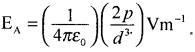Question 28.
Mention the method of electrification of silk with glass rod.
Frictional method.

Question 29.
What helps the electrification of insulators?
Due to friction between the two insulators, heat is generated. This heat ionises the insulators.

Question 30.
Give the sign convention for charge as given by Benjamin Franklin.
When glass rod is rubbed with silk, the nature of charge on the glass rod is taken as positive. and that on silk is taken as negative.

Question 31.
Name the apparatus to detect the charge on a body.
Gold Leaf Electroscope.Question 32.
Mention any one method of charging a body
A body can be charged by the method of friction.
Note : The other two types of charging a body are

1. conduction
2. induction.

Question 33.
Can an insulator be charged by the method of conduction?
No, only conductors can be charged by the method of conduction.

Question 34.
Can a metal be charged by induction ?
Yes.

Question 35.
What are point charges?
If the linear size of the charged bodies are much smaller than their distance of separation, then these charged bodies are called point charges, e.g.: Electrons, protons, ions, molecules.

Question 36.
Mention the value of electrical permittivity of free space.
ε0 = 8.854 × 10-12 Fm-1 or C2N-1m-2.

Question 37.
Give the mathematical form of the combined forces acting on the given charge due to a number of discrete point charges.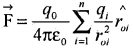Question 38.
Express electric force in terms of electric field intensity.Question 39.
What is the direction of electric field due to a positive charge?
The direction of electric field is radially outward due to a positive charge.

Question 40.
What is the direction of electric field due to a negative charge?
The direction of electric field is radially inward due to a negative charge.

Question 41.
What is the amount of electric flux through a closed surface due to an electric dipole?
Zero.

Question 42.
Give the mathematical form of Gauss law in electrostatics.
Φ = $$\frac{1}{\varepsilon_{0}}$$(q1 + q2 + …. + qn)

Question 43.
Mention the SI unit of electric flux.
Vm (volt metre).Question 44.
Define linear charge density.
The ratio of charge on a body to its length is known as linear charge density.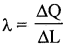Question 45.
Define surface charge density.
The ratio of charge on a body to its surface area is known as surface charge density.Question 46.
Define volume charge density.
The rãtio of charge on a body to its volume is known as volume charge density.Question 47.
What is electrostatic shielding? Mention its one application.
Electric lines of force do not pass through a metallic conductor. This property is known as Electrostatic shielding. This property is used to prevent tuner circuit from straight electric and magnetic fields.

Question 48.
What is the electric field intensity at the centre of a charged spherical shell. ‘
Zero.

Question 49.
Does charge on a body vary under rotation and frame of reference in relative motion.
No. It is invariant of frame of reference and under rotation.

Question 50.
Say whether electric field is a continuous function across the surface or not.
No. Electric field is discontinuous across the surface.

Question 51.
Represent area element in a vector form.is the magnitude of the area element and n̂ is normal to the area element.

Question 52.
Is Gauss’s law true for any shape and size of a closed surface?.
Yes. Gauss’s law is true for any closed surface.Question 53.
A spherical surface encloses a net charge ‘q’ and hence TOEF through the surface is $$\left(\frac{q}{\varepsilon_{0}}\right)$$ If the spherical surface is replaced by a cubical surface then what will be the TOEF through it?
Same as Φ = $$\frac{q}{\varepsilon_{0}}$$
Note :

•  TOEF through any one surface of a cube = $$\frac{q}{6 \varepsilon_{0}}$$
•  TOEF through the edges of a cube = $$\frac{q}{12 \varepsilon_{0}}$$

Question 54.
Mention the value of Electric field intensity near the earth’s surface.

Question 55.
Define S.I. unit of charge. (March 2015)
One coulomb of charge is that charge which when placed in air/vacuum at a distance of 1 m from an identical charge experiences a force of repulsion of magnitude 9 × 109N.

### 2nd PUC Physics Electric Charges and Fields Two Marks Questions and Answers

Question 1.
State Coulomb’s law in electrostatics. Give the expression for force in scalar form. (March 2014)
Coulomb’s law: ‘The Electrostatic force of attraction or repulsion between two stationary point charges separated in free space or air is directly proportional to the product of magnitudes of charge on them and inversely proportional to the square of the distance between them and the force acts along the line joining the two point charges’.Question 2.
Give the vector form of Coulomb’s law. (March 2015)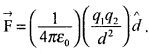Where, d̂ is the unit vector along the direction of force.

Question 3.
Give the expression for electrostatic force in position vector form. (March 2015)Question 4.
How many electrons are transferred to a body in order to charge it to -5C?
One coulomb of charge is associated with 6.25 × 1018 electrons.
Therefore for – 5C of charge 6.25 × 1018 × 5 = 3.125 × 1019 electrons have to be transferred.Question 5.
Represent vectorially the direction of force between the charges for
(i) q1q2 > 0
(ii) q1q2 < 0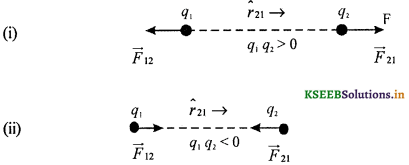Question 6.
Write the expression for the electric field at a point on the perpendicular bisector due to a short electric dipole. Give the meaning of the symbols used.where p – electric dipole moment, d- distance.

Question 7.
Write the expression for the electric field at any point due to a short electric dipole. Give the meaning of the symbols used.where p – electric dipole moment, d- distance.
θ – angle between the dipole axis and line joining the point and the centre.

Question 8.
Write the expression for torque experienced by an electric dipole in an uniform electric field. Give the meaning of the symbols used.
Torque τ = pE sin θ where ‘θ’ is the angle between the dipole axis and the direction of uniform Electric field, p – electric dipole moment and E – uniform electric field intensity.

Question 9.
Why is that gravitational force negligible compared to the electrostatic force between a proton and an electron? (Assume the distance between them as 1 Å )
The acceleration of electron will be 2.5 × 1022 ms-2 and that of proton 1.4 × 1019 ms-2. The acceleration due to gravity is 10ms~ which is far less than what arises due to Coulombian force.

Question 10.
State the principle of superposition of forces acting on a test charge.
If the force on any charge due to a number of other charges is independent of the presence of other charges, then the net force is the vector sum of the forces acting on the test charge taken independently.

Question 11.
Give the expression for electric field intensity at a point due to a thin infinitely long straight wire. Give the meaning of the
$$\vec{E}$$ = $$\frac{\lambda}{2 \pi \varepsilon_{0} r} \hat{n}$$ where n̂ is the unit vector in the direction of $$\vec{E}$$ and λ, is the uniform linear charge density.Question 12.
Give the expression for electric field intensity due to an infinite thin plane sheet. Give the meaning of the symbols used.
$$\vec{E}$$ = $$\frac{\sigma}{2 \varepsilon_{0}} \hat{n}$$ where n̂ unit vector normal to the plane, outward on either side, σ – uniform surface charge density.

Question 13.
Write the expression for electric field intensity at any point outside and inside due to a charged spherical shell.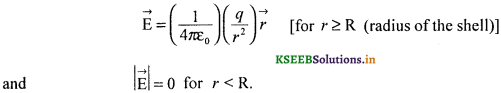Question 14.
Why ¡s an atom electrically neutral?
The number of positive charges in the nucleus of an atom, balances the number of negative charges revolving around the nucleus. Hence on atom is electrically neutral.

Question 15.
Give the expression for torque experienced by an electric dipole in an uniform electric field in a vector form. Give the meaning of the symbols used.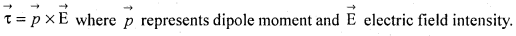Question 16.
Why is that electric field intensity at a point on the equatorial line due to an electric dipole taken as negative?
The direction of $$\vec{E}$$ and $$\vec{P}$$ (displacement moment) act opposite to each other and hence, electric field intensity is taken as negative.

Question 17.
Give the expression for electric field intensity at a point due to a discrete and continuous distribution of charges.Question 18.
Do electrostatic field lines form (electrostatic) closed loops? Give reason.
Electrostatic field lines do not form any closed loops. This is due to the conservative nature of electric field.

Question 19.
An electrically charged oil drop remains static at a point in an electric field. Represent the situation by a mathematical equation.
mg = $$\frac{q \mathrm{V}}{d}$$
where V – electric potential difference between the charged plates,
d – is the distance between the plates.
m – mass of oil drop
g – acceleration due to gravity
q – electric charge on the oil drop.Question 20.
Why is electrostatic force conservative and central?
Electrostatic force is conservative because the work done by the force depends on the initial and the final points of a test charge.
Electrostatic force is central because the force acts along the line joining the centres of these charges.

Question 21.
Calculate the number of electrons to be transferred from a material body in order to charge it to + 5.5 nC.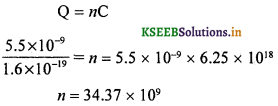Question 22.
Show graphically : E ∝ $$\frac{1}{r^{2}}$$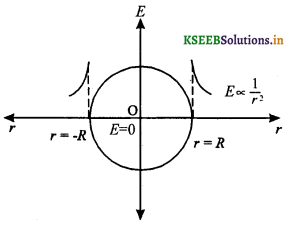### 2nd PUC Physics Electric Charges and Fields Three Marks Questions and Answers

Question 1.
Represent electric field lines around
(i) a positive point charge
(ii) a negative point charge and
(iii) an electric dipole.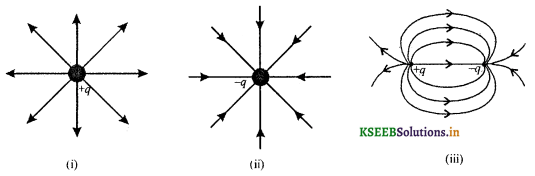Question 2.
Mention any three properties of an electric charge. (July 2014)
(a) Charge is conserved and universal
(b) Charge is quantised
(d) Charge is invariant of speed.

Question 3.
Write any three characteristics of electrostatic force.
(a) Electrostatic force is a central, conservative and long range force.
(b) Electrostatic force is both an attractive and a repulsive force.
(c) Electrostatic force is a non contact force and is 1039 times stronger than gravitational force and one hundredth of nuclear force.Question 4.
What decrease of mass of a body is observed when charged to +10C?
1C = 6.25 × 1018 electron transfer
∴ 10C results in a transfer of 62.5 × 1018 electrons
mass of electrons transferred = (62.5 × 1018) (9.1 × 10-31kg) = 5.688 × 10-11 kg.
∴ The decrease of mass of a body is 5.688 × 10-11 kg.

Question 5.
If the distance between the two point charges is doubled then what will be percentage change in the force?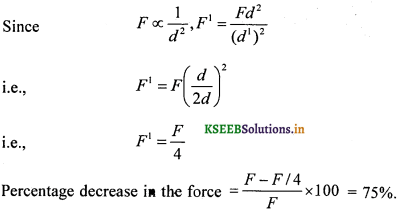Question 6.
The sum of charge on two point charges is ‘Q’. What should be the values of chargeson them for a maximum force between them for a given separation?
Given q1 + q2 = Q
or q2 = Q – q1
Force between the two point chargesFor a maximum force,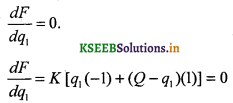i.e., -q1 + Q – q1 = 0
or Q = 2q1
∴ q1 = $$\frac{Q}{2}$$ and q2 = $$\frac{Q}{2}$$Question 7.
For a given separation between the two point charges, the force between them is 64 × 10-4 N. If the distance is decreased by 0.25 m then the force between them ¡s 1oo × 10-4 N. Find the initial separation between the charges.Question 8.
Represent the force between the two point charges in terms of position vector graphically.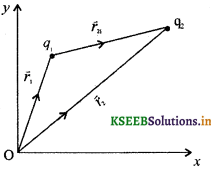Question 9.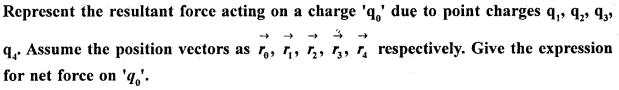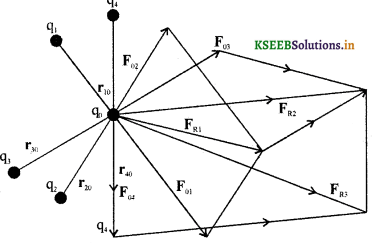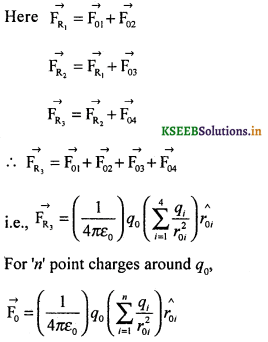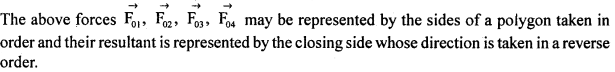Question 10.
Represent the electric field intensity at a point (where a unit positive charge is assumed to be) due to a system of charges.
In the above figure, replace ‘q0‘ by + 1C and $$\vec{F}$$ by $$\vec{E}$$P is at the origin, its coordinate may be taken as (0,0).

Question 11.
Represent pictorially how electric flux may be taken as the dot product of $$\vec{E}$$ and $$\Delta \vec{s}$$.If S1 makes an angle with S2 then their normals at the common centre makes an angle of 0.
The component of $$\vec{E}$$ along the normal at the new inclination will be Ecosθ.
We note that, ΔΦ = (Ecosθ)Δs= EΔScosθ = $$\overrightarrow{\mathrm{E}} \cdot \Delta \vec{s}$$
Hence electric flux ΣΔΦ = Σ$$\overrightarrow{\mathrm{E}} \cdot \Delta \vec{s}$$

Question 12.
State and explain Gauss’s theorem in Electrostatics.
Statement: ‘The total outward Electric flux in air through any closed surface is equal to (1/εo) times the total charge enclosed by it’.
Let q1, q2, q3 ……. qn be the charge enclosed by a system of discrete charges.
According to gauss theorem‘Σds’ is the effective area through which component of Electric field ‘E cos θ’ does not vanish.### 2nd PUC Physics Electric Charges and Fields Five Marks Questions And Answers

Question 1.
State Gauss.’s theorem. Obtain an expression for electric intensity at a point due to a charged spherical hollow conductor (shell). (March 2014, July 2014)Gauss’s theorem: ‘The total outward electric flux passing through a closed surface in air is $$\left(\frac{1}{\varepsilon_{0}}\right)$$ times the total charge enclosed by it’.
I7 ¡L .
L,et ‘+Q’ be the charge enclosed by a hollow conductor of radius ‘R’.
Let ‘p’ be a point at a distance V from the centre of the conductor Let‘efe’ be a small element of area surrounding the
point. A normal drawn from the point coincides with the direction of $$\vec{E}$$ . Hence cos 0° = 1. From Gauss’theoremThis electric field intensity is maximum. Since electric flux depends on the charge enclosed, and electric field intensity depends on the electric flux, electric field remains zero at all points inside the spherical hollow conductor.

Question 2.
Mention any five properties of electric field lines.

1. Electric lines of force never intersect with each other.
2. Electric lines of force exert lateral stress on one another.
3. Electric lines of force do not enter a conductor.
4. The direction of electric field is given by the direction of tangent drawn at that point on an electric line of force.
5. In the case of uniform electric field, the electric lines of force remain parallel with each other.
6. Electric flux per unit surface area normal to the flux determines the electric field intensity.Question 3.
Obtain an expression for torque experienced by an Electric dipole.
Force on ‘-q’ of an electric dipole due to on electric field ‘E is ‘-qE’ and on ‘+q’ is ‘+qE These two forces are equal and opposite and act at ‘A’ and ‘B’ on the dipole. These forces constitute a restoring couple and maintains the position of the dipole in the field.Torque τ = (Force) (arm of the couple)
i.e., τ = F $$\overline{\mathrm{AC}}$$
i.e., τ = F $$\overline{\mathrm{AB}}$$ sin θ
i.e., τ = (qE) (21) sin θ
i.e., τ = pE sin θ
Where, p = 2lq is called dipole moment.
$$\vec{τ }$$ = pE sin θ n̂
where, n̂ is unit vector along $$\vec{t}$$
also $$\vec{\tau}=\vec{p} \times \overrightarrow{\mathrm{E}}$$

Question 4.
Obtain an expression for the electric field intensity at a point due to an infinitely long straight uniformly charged wire.Let the Gaussian surface be taken as a cylinder of length / and radius r. Electric field lines drawn from a point on the wire to every point on the rim of a circular plane make an angle of zero with respect to the normal drawn to the surface (fig (a)).
Electric flux through the Gaussian surface = E(SA); SA = surface area
= E(2πrl)
If λ represents linear charge density of the thin wire, then total charge Σq on the wire = λl.
From Gauss Law, total outward electric flux through a closed surface = $$\frac{1}{\varepsilon_{0}}$$ (total charge enclosed)For an infinitely long wire, Φ1 = Φ2 = 90°, the magnitude of electric field E = $$\frac{\lambda}{2 \pi \varepsilon_{0} r}$$
where, sinΦ1 + sinΦ2 = 2Question 5.
Obtain an expression for the electric field intensity at a point on the dipole axis.
Let +1C be the test charge at P, at a distance r from the centre of an electric dipole.
Test charge + 1C experiences a force of repulsion along BP due to +q at B and attraction due to -q along PA.
Since ‘+q’ is nearer than -q from P, the resultant force points along BP.
Force experienced by a unit +ve charge is called electric field.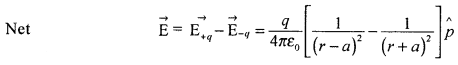where, P̂ represents the unit vector of dipole moment point from – q to + q.Question 6.
Obtain an expression for the electric field intensity at a point on the equatorial line of an electric dipole.
Let q be the magnitude of charge on the dipole. Let 2a be the dipole length. Let r be the distance of a point from the centre of the dipole. Let PO be perpendicular to AB. The direction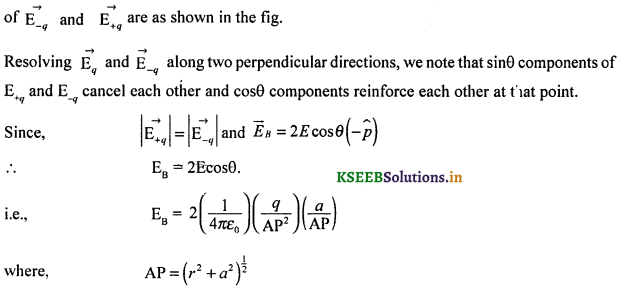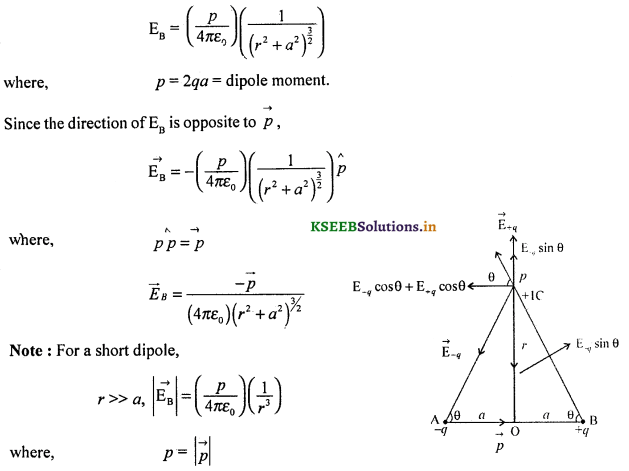Question 7.
Obtain an expression for the electric field intensity due to a uniformly charged infinite plane sheet.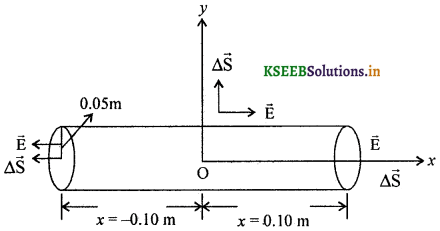Let σ be the surface charge density, $$\vec{E}$$ be the electric field intensity. Let A be the cross-sectional area of G.S.
Flux across the base areas will be the same.
Net flux through them = 2EA cosθ where θ = cos0° = 1.
i.e., Φ = 2EA
From Gauss’ law,
TOEF = Φ = $$\frac{1}{\varepsilon_{0}}$$ (total charge on the plate)
i.e., 2EA = $$\frac{1}{\varepsilon_{0}}$$ (σA)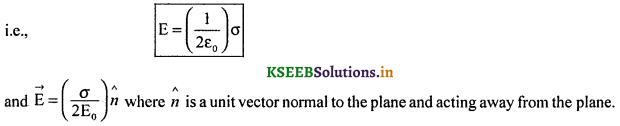### 2nd PUC Physics Electric Charges and Fields List of Formulae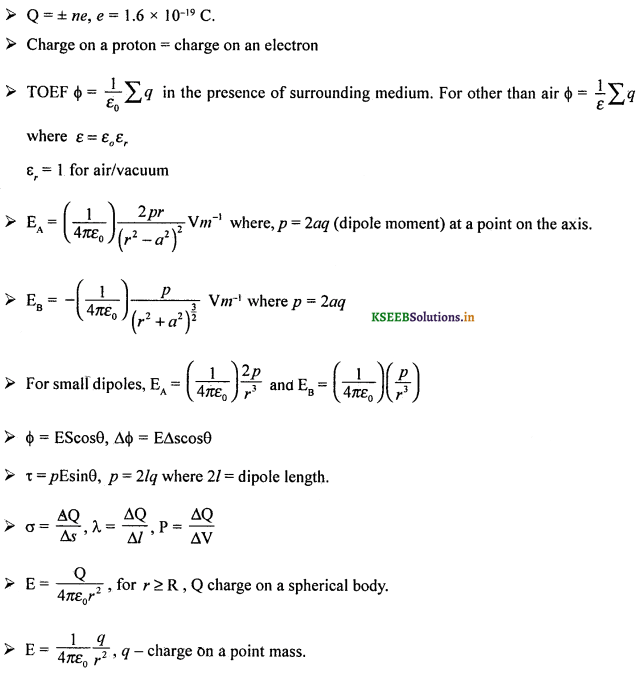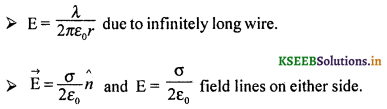### 2nd PUC Physics Electric Charges and Fields Numericals and Solutions

Question 1.
Two point charges-2nC and + 8nC are separated in air by a distance of 0.10 m. Find the position of the neutral point along the line joining the 2 point charges.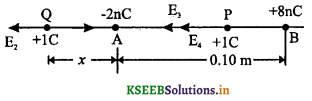q1 = -2nC, q2 = +8nC, d = 0.10 m
E1 – E2 = 0 at Q, E3 + E4 ≠ 0 at P
E1 = E2 (for neutral point)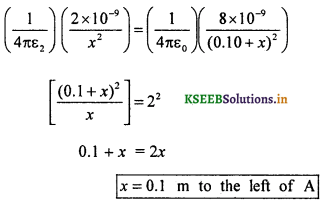= 22

Question 2.
Two point charges q1 = + 4μc & q2 = + 32μc are separated in air at a distance of 0.12m apart. Find the position of the neutral point along the line joining the 2 point charges.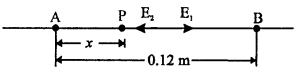q1 = + 4μc, q2 = + 32μc d= 0.12 m
For neutral point E1 = E2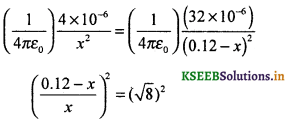Question 3.
Two point charges + 10 μC and -15 μC are placed at the vertices A and B of a right angle ∆leABC, such that AB = 0.05 m, BC = 0.12 m. Calculate the resultant field intensity at the point C. If + 100μC of charge is placed at C, then what force will be experienced by the point charge at C?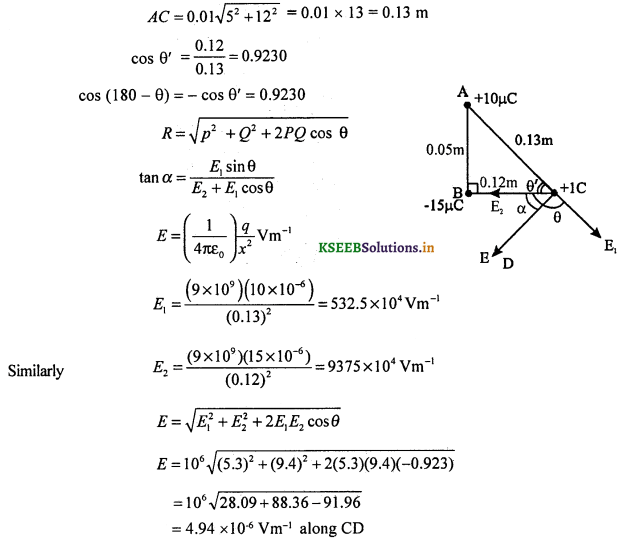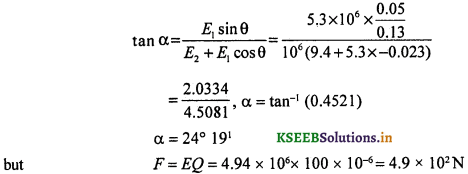Question 4.
Four point charges + 2nC, – 3nC, + 4nC, – 5nC are placed at the vertices A, B, C, D of a square ABCD of side 0.2 m respectively. Calculate the electric field intensity at a point of intersection of the diagonals.
The angles between E1 and E2, E3 and E4 and E5 and E6, θ = 90°
AC = (√2) (0.2) m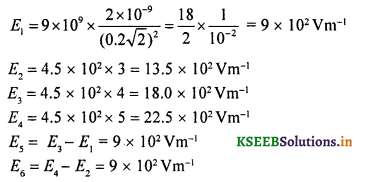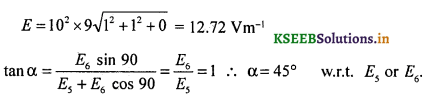Question 5.
In the gåvcn figure, what is the Flux enclosed by A and B?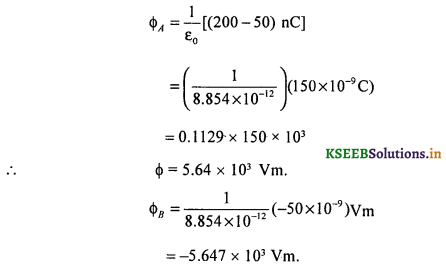Question 6.
A system has two charges qA = 2.5 × 10-7 C and qB = -2.5 × 10-7C located at points A = (0, 0, – 15 cm) and B = (0, 0, + 15cm) respectively. What are the total charge and electric dipole moment of the system?
(a) Net charge of the system = q = zero.The distance between the dipoles = 2(15) = 30 cm = 0.30 m
Dipole moment = 2aq
= 0.30 × 2.5 × 10-7
= 0.75 × 10-7 Cm
= 7.5 × 10-8 Cm along the z-axis.

Question 7.
Two point charges + 10 nC and -10 nC are separated by a distance of 0.02m. Calculate the electric field intensity at a point P on the axis of the dipole at 0.02 from + 10nC.Question 8.
An electric dipole with dipole moment 4 × 10-9 cm is aligned at 30° with the direction of a uniform electric field of magnitude 5 x 104 NC_1. Calculate the magnitude of the torque acting on the dipole.Given p = 4 × 10-9 Cm, θ = 30°
E = 5 × 104 NC-1
torque τ = pE sin θ
i.e., τ = 4 × 109 × 5 × 1o-4 × sin 30°
i.e., τ = 1 × 10-4 Nm
Hence torque acting on the system = 1.0 × 10-4Nm.Question 9.
(a) Two insulated charged copper spheres A and B have their centres separated by a distance of 0.5m. What is the mutual force of electrostatic repulsion if the charge on each is 6.5 × 10-7 C? Assume that the radii of A and B are negligible compared to the distance of separation.
(b) What is the force of repulsion if each sphere is charged double the above amount and the distance between them is halved?
Given r = 0.5m, qA = qB = 6.5 × 10-5 C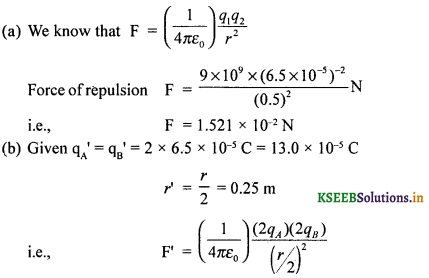i.e., F’ = 16F = 16 × 1.521 × 10-2N = 24.336 × 10-2 N = 0.24N
The new force is sixteen times the old and is repulsive.

Question 10.
Two insulated charged copper spheres A and B have their centres separated by a distance of 0.5 m. The charge on each sphere is 6.5 × 10-7 C. A third sphere ‘C’ of identified! shape and size is brought in contact with ‘A’ and them brought in contact with B and finally removed. What is the new force between A and B?
Initial charge on A and B; qA = qB = 6.5 × 10-7 C
When an uncharged conductor ‘C is brought in contact with ‘A’, then charge on A and C will be 3.25 × 10-7 C. When ‘C’4s brought in contact with B,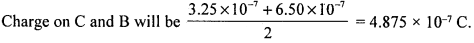The force of repulsion between 3.25 × 10-7 C (on A) andThe new force between A and B is 5.7 × 10-3 N

Question 11.
Consider a uniform electric field $$\vec{E}$$ = 3 × 103 î NC-1
(a) What is the flux of this Held through a square of 0.1m on a side whose plane is parallel to the y-z plane?
(b) What is the flux through the same square if the normal to its plane makes a 60 angle with x-axis?
Given $$\vec{E}$$ = 3 × 103 î NC-1
(a) A normal drawn from ‘P’ will coincide with $$\vec{E}$$.
Using the formule for electric flux,
Φ = ES cos θ ; θ = 0°
i.e., Φ = ES = 3 × 103 × (0.1)2 Vm
i.e., Φ – 30 Vm (or Nm2 C-1)

(b) For θ = 60°,
Φ = 3 × 103 × (0.1)2 × cos 60°
i.e., Φ = 15 Vm (or Nm2 C-1)Question 12.
A uniformly charged conducting sphere of 2.4 m diameter has a surface charge density of 80 × 10-6 cm-2.
(a) Find the charge on the sphere.
(b) What is the total electric flux leaving the surface of the sphere?
Given diameter d = 2.4m
surface charge density σ = 80 × 10-6 cm-2

(a) but σ = $$\frac{\theta}{4 \pi r^{2}}$$
∴ Q = (4πr2
= 4 × 3.142 × (1.2)2 × 80 × 10-6 C
Q = 1.45 × 10-3 C

(b) Electric flux Φ = $$\frac{Q}{\varepsilon_{0}}$$
i.e., Φ = $$\frac{1.45 \times 10^{-3}}{8.854 \times 10^{-12}}$$
i.e., Φ = 1.64 × 108 Vm (or Nm2 C-1)
Flux leaving the surface = 1.6 × 108 Vm.

Question 13.
An infinite line charge produces a field of 9 × 104 NC-1 at a distance of 2 × 10-2 m. Calculate the linear charge density.
Given: E = 9 × 104 NC-1
r = 2 × 10-2 m
We Know E = $$\frac{\lambda}{2 \pi \varepsilon_{0} r}$$
i.e., λ = 2πε0rE where λ – is linear charge density.
i.e., λ = 2 × 3.142 × 8.854 × 10-12 × 2 × 10-2 × 9 × 104 Cm-1
i.e., λ = 1 × 10-7 Cm-1
Linear charge density 1 × 10-7 Cm-1

Question 14.
Two large, thin metal plates arc parallel and close to each other. On their inner faces, the plates have surface charge densities of opposite signs and magnitude 17.0 × 10-22 Cm-2. what is the electric field
(a) in the outer región of the first and the second plates?
(b) between the plates?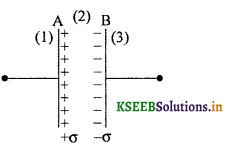(a) Electric field in the region (1) ¡s(b) Electric field in the region (3) ¡s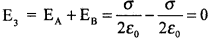(c) Electric field in the region (2) ¡s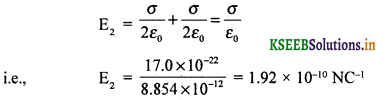Thus Electric field in between the plates = 1.92 × 10-10 NC-1Question 15.
An oil drop of 12 excess electrons is held stationary under a constant electric field of 2.55 × 104NC-1 in Millikan’s oil drop experiment. The density of the oil is 1.26 × 103 kgm-3. Estimate the radius of the drop (g = 9.81 ms 2, e = 1.60 x 10-19 C).
Given charge on an oil drop
q = 12e = 12 × 1.60 × 10-19C
i.e., q = 19.20 × 10-19C .
E = 2.55 × 104 NC-1
p = 1.26 × 103 kgm-3mass = m = (p) (volume)
i.e., m = (4/3) πr3p
i.e., m = $$\frac { 4 }{ 3 }$$ × .3.142 × r3 × 1.26 × 103 kg
i.e., m = 5.278 (r3) × 103 kg
given gravitational force = electrostatic force
i.e., mg = qE
i.e., 103 × 5.278 r3g = 19.2 × 10-19 × .2.55 × 104
i.e., r3 = $$\frac{48.96 \times 10^{-15}}{10^{3} \times 5.278 \times 9.81}$$ = 0.9455 × 10-18
i.e, r ≈ 0.981 × 10-6 m
or r = 9.81 × 10-7 m
Thus, the radius of the drop is 9.81 × 10-7 m

Question 16.
In a certain region of space, electric field is along the z-direction throughout. The magnitude of electric field is, however not constant but increases uniformly along the positive z-direction at the rate of 105 NC-1m-1. What are the force and torque experienced by a system having a total dipole moment equal to 10-7 Cm in the negative z-direction?
Given $$\frac{\mathrm{dE}}{\mathrm{dz}}$$ = 105 NC-1m-1
Then F = qdE, represents the force on the system.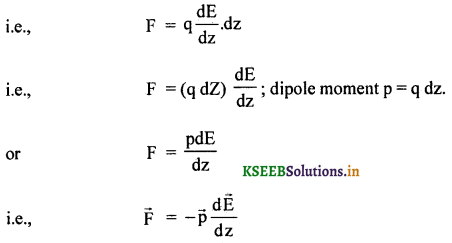∴ F = -10-7 × 105N
i.e., F = -10-2 N.
Force on the dipole is -10-2 N
Now the angle between the dipole and the electric field is 180°.
Torque τ = pE sin θ.
i.e., τ = -10-7 × 10-2 × sin 180°
i.e., τ = 0
Thus the torque on the dipole ¡s zero.Question 17.
The electric field components are Ex = ax1/2, Ey = Ez= 0 in which a = 800 NC-1 m-1/2. Calculate
(a) the flux through the cube, and
(b) the charge within the cube. Assume that a = 0.1 m.Given Eleft end = αx1/2 = αa1/2 where x = a
ERight end = α(2a)1/2
Flux = ΦLeft face = EL ∆S cos θ = EL ∆S cos 180°
through left face
= -EL ∆S = -ELa2
similarly ΦRight face = ER ∆S cos ° = ER a2
Net electric flux Φ = ΦL + ΦR
= a2 (ER + EL)
= a2(21/2 αa2 – αa1/2)
= αa5/2(√2 – 1)
but a = 800 NC-1m-1/2, a = 0.1m
∴ Electric flux Φ = (800)(0.1)5/2(√2 – 1)
= 1.05 Vm (or Nm2 C-1)

(b) Net charge q = ε0Φ = 8.854 × 10-12 × 1.05 C
= 9.27 × 10-12 CQuestion 18.
An electric field is uniform and E = 200 î NC-1 along + x and E = -200 î NC-1 along —JC direction. A right circular cylinder of length 0.20 m and radius 0.05m has its centre at the origin and its axis along the x-axis so that one face is at x = +0.10m and the other x = -0.10m.
(a) What is the net outward flux thorugh each flat face?
(b) What is the flux through the side of the cylinder?
(c) What is the net outward flux throught the cylinder?
(d) What is the net charge inside the cylinder?(a) Electric flux through the left flat face
ΦL = EL∆S cos θ ; θ = 180° ; cos 180° = -1
Hence ΦL = -200 × 3.142 × (0.05)2 × -1
i.e., ΦL = +1.57 Vm (or Nm2 C-1)
Electric flux through the right flat face,
ΦR = ER ∆S cos θ ; θ = 0°; cos 0° = +1
i.e., ΦR = 200 × 3.142 × (0.05)2 × 1
i.e., ΦR = +1.57 Vm (or Nm2 C-1)

(b) Electric flux through the canvas or curved surface area,
ΦCSA = E ∆S cos 90° = 0

(c) Net outward flux through the cylinder, Φ = 1.57 + 1.57 + 0 = 3.14 Nm2 C-1

(d) .The net charge within the cylinder can be found by using the relation q = e0Φ
i.e., q = 8.854 × 1o-12 × 3.14 C
or q = 2.78 × 10-11 C